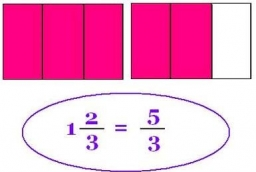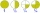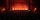# Simplify expression with mixed

When (7¹/3 + 2¾)÷(2 + 2¼ × 1¹/3) is simplified the result is?

x =  2.0167

### Step-by-step explanation:Did you find an error or inaccuracy? Feel free to write us. Thank you!Tips to related online calculators
Need help to calculate sum, simplify or multiply fractions? Try our fraction calculator.

## Related math problems and questions:

• Simplify 7Simplify. 7-1/3÷ 3-2/3 of 2+ 4-1/2÷ 2-1/4+ 1/2 solution and by step by step
• Fractions and mixed numerals(a) Convert the following mixed numbers to improper fractions. i. 3 5/8 ii. 7 7/6 (b) Convert the following improper fraction to mixed number. i. 13/4 ii. 78/5 (c) Simplify these fractions to their lowest terms. i. 36/42 ii. 27/45 2. evaluate following ex
• Simplify 3Simplify mixed numerals expression: 8 1/4- 3 2/5 - (2 1/3 - 1/4) Show your solution.
• Evaluate fractionsThe difference  of 5 1/2 and 2/3 is added to the product of 5/6 and 1/2
• Evaluate mixed expressionsWhich of the following is equal to 4 and 2 over 3 divided by 3 and 1 over 2? A. 4 and 2 over 3 times 3 and 2 over 1 B. 14 over 3 times 2 over 7 C. 14 over 3 times 7 over 2 D. 42 over 3 times 2 over 31
• Sum of 18Sum of two fractions is 4 3/7. If one of the fractions is 2 1/5 find the other one .
• Equivalent fractions 2Write the equivalent multiplication expression. 2 1/6÷3/4
• Equation with mixed 2A number, X, is subtracted from 8 1/4. The result is 12 3/5. What is the value of X?3 3/4 + 2 3/5 + 5 1/2 Show your solution.
• Rose spendsRose spends 2 1/3 hours studying Math, 1 3/4 hours studying English, and 2 1/4 hours studying Science. Find her average time studying the three subjects.
• The product 2The product of two functions is 10. If one of them is 2 1/3, find the other one.
• Product increasedWhen the product of 2/3 and 6/10 is increased by 2/5, the result is?
• The sum 12The sum of 3 mixed numbers is 20 13/15. two of the numbers are 6 1/3 and 7 5/6. what is the third number?
• Evaluate expressionEvaluate expression using BODMAS rule: 1 1/4+1 1/5÷3/5-5/8
• A large 2A large popcorn bag holds four times as much as a small popcorn bag at the end of the party 3 1/3 small bags and 2 1/4 large bags left. How many small bags with the leftover popcorn fill?
• Golf ballWhen a golf ball is hit with a 2 iron, it rotates at a rate of 8 1/3 times/second. How many times does this ball rotate if it is airborn for 7 1/3 seconds
• Numbers divisionWith what number should be divided mixed number 2 3/4 to get 11/12?